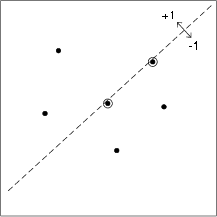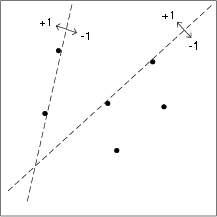# 0.3 Introduction to complexity regularization  (Page 3/3)

 Page 3 / 3

The number of unique labellings of the training data that can be achieved with linear classifiers is, in fact, finite. A line can bedefined by picking any pair of training points, as illustrated in [link] . Two classifiers can be defined from each such line: one that outputs a label “1” for everything on or abovethe line, and another that outputs “0” for everything on or above. There exist $\left(\genfrac{}{}{0pt}{}{n}{2}\right)$ such pairs of training points, and these define all possible unique labellings of the training data.Therefore, there are at most $2\left(\genfrac{}{}{0pt}{}{n}{2}\right)$ unique linear classifiers for any random set of $n$ 2-dimensional features (the factor of 2 is due to the fact that for each linear classifier thereare 2 possible assignments of the labelling).Fitting a linear classifier to 2-dimensional data. There are an infinite number of such classifiers. We can generate alinear classifier by choosing two data points, drawing a line with both points on one side, and declaring all points on or above theline to be “ + 1 ” (or “ - 1 ”) and all points below the line to be “ - 1 ” (or “ + 1 ”).From the discussion in the previous figure, we see that the two linear classifiers depicted in this figure are equivalent for this setof data points, and hence relative to the set of n training data there are only on the order of n 2 unique linear classifiers.

Thus, instead of infinitely many linear classifiers, we realize that as far as a random sample of $n$ training data is concerned, there are at most

$\begin{array}{ccc}\hfill 2\left(\genfrac{}{}{0pt}{}{n}{2}\right)& =& \frac{2n!}{\left(n-2\right)!2!}\hfill \\ & =& n\left(n-1\right)\hfill \end{array}$

unique linear classifiers. That is, using linear classification rules, there are at most $n\left(n-1\right)\approx {n}^{2}$ unique label assignments for $n$ data points. If we like, we can encode each possibility with ${log}_{2}n\left(n-1\right)\approx 2{log}_{2}n$ bits. In $d$ dimensions there are $2\left(\genfrac{}{}{0pt}{}{n}{d}\right)$ hyperplane classification rules which can be encoded in roughly $d{log}_{2}n$ bits. Roughly speaking, the number of bits required for encoding each model is the VC dimension. Theremarkable aspect of the VC dimension is that it is often finite even when $\mathcal{F}$ is infinite (as in this example).

If $\mathcal{X}$ has $d$ dimensions in total, we might consider linear classifiers based on $1,2,\cdots ,d$ features at a time. Lower dimensional hyperplanes are less complex than higher dimensionalones. Suppose we set

$\begin{array}{ccc}\hfill {\mathcal{F}}_{1}& =& \text{linear}\phantom{\rule{4.pt}{0ex}}\text{classifiers}\phantom{\rule{4.pt}{0ex}}\text{using}\phantom{\rule{4.pt}{0ex}}\text{1}\phantom{\rule{4.pt}{0ex}}\text{feature}\hfill \\ \hfill {\mathcal{F}}_{2}& =& \text{linear}\phantom{\rule{4.pt}{0ex}}\text{classifiers}\phantom{\rule{4.pt}{0ex}}\text{using}\phantom{\rule{4.pt}{0ex}}\text{2}\phantom{\rule{4.pt}{0ex}}\text{features}\hfill \\ \hfill \cdots & & \text{and}\phantom{\rule{4.pt}{0ex}}\text{so}\phantom{\rule{4.pt}{0ex}}\text{on}\phantom{\rule{4.pt}{0ex}}\hfill \end{array}.$

These spaces have increasing VC dimensions, and we can try to balance the empirical risk and a cost function depending on the VC dimension.Such procedures are often referred to as Structural Risk Minimization . This gives you a glimpse of what the VC dimension is all about. In future lectures we will revisit this topic in greaterdetail.

## Hold-out methods

The basic idea of “hold-out” methods is to split the $n$ samples $D\equiv {\left\{{X}_{i},{Y}_{i}\right\}}_{i=1}^{n}$ into a training set, ${D}_{T}$ , and a test set, ${D}_{V}$ .

$\begin{array}{ccc}\hfill {D}_{T}={\left\{{X}_{i},{Y}_{i}\right\}}_{i=1}^{m},& & {D}_{V}={\left\{{X}_{i},{Y}_{i}\right\}}_{i=m+1}^{n}\hfill \end{array}.$

Now, suppose we have a collection of different model spaces $\left\{{\mathcal{F}}_{\lambda }\right\}$ indexed by $\lambda \in \Lambda$ (e.g., ${\mathcal{F}}_{\lambda }$ is the set of polynomials of degree $d$ , with $\lambda =d$ ), or suppose that we have a collection of complexity penalization criteria ${L}_{\lambda }\left(f\right)$ indexed by $\lambda$ ( e.g., let ${L}_{\lambda }\left(f\right)=\stackrel{^}{R}\left(f\right)+\lambda c\left(f\right)$ , with $\lambda \in {\mathbf{R}}^{+}$ ). We can obtain candidate solutions using the training set as follows. Define

$\begin{array}{ccc}\hfill {\stackrel{^}{R}}_{m}\left(f\right)& =& \sum _{i=1}^{m}\ell \left(f\left({X}_{i}\right),{Y}_{i}\right)\hfill \end{array}$

and take

$\begin{array}{ccc}\hfill {\stackrel{^}{f}}_{\lambda }& =& arg\underset{f\in {\mathcal{F}}_{\lambda }}{min}{\stackrel{^}{R}}_{m}\left(f\right)\hfill \end{array}$

or

$\begin{array}{ccc}\hfill {\stackrel{^}{f}}_{\lambda }& =& arg\underset{f\in \mathcal{F}}{min}\phantom{\rule{0.166667em}{0ex}}\left\{{\stackrel{^}{R}}_{m},\left(f\right),+,\lambda ,c,\left(f\right)\right\}\hfill \end{array}.$

This provides us with a set of candidate solutions $\left\{{\stackrel{^}{f}}_{\lambda }\right\}$ . Then we can define the hold-out error estimate using the test set:

$\begin{array}{ccc}\hfill {\stackrel{^}{R}}_{V}\left(f\right)& =& \frac{1}{n-m+1}\sum _{i=m+1}^{n}\ell \left(f\left({X}_{i}\right),{Y}_{i}\right),\hfill \end{array}$

and select the “best” model to be $\stackrel{^}{f}={\stackrel{^}{f}}_{\stackrel{^}{\lambda }}$ where

$\begin{array}{ccc}\hfill \stackrel{^}{\lambda }& =& arg\underset{\lambda }{min}{\stackrel{^}{R}}_{V}\left({\stackrel{^}{f}}_{\lambda }\right)\hfill \end{array}.$

This type of procedure has many nice theoretical guarantees, provided both the training and test set grow with $n$ .

## Leaving-one-out cross-validation

A very popular hold-out method is the so call “leaving-one-out cross-validation” studied in depth by Grace Wahba (UW-Madison,Statistics). For each $\lambda$ we compute

$\begin{array}{ccc}\hfill {\stackrel{^}{f}}_{\lambda }^{\left(k\right)}& =& arg\underset{f\in \mathcal{F}}{min}\frac{1}{n}\sum _{\stackrel{i=1}{i\ne k}}^{n}\ell \left(f\left({X}_{i}\right),{Y}_{i}\right)+\lambda C\left(f\right)\hfill \end{array}$

or

$\begin{array}{ccc}\hfill {\stackrel{^}{f}}_{\lambda }^{\left(k\right)}& =& arg\underset{f\in {\mathcal{F}}_{\lambda }}{min}\frac{1}{n}\sum _{\stackrel{i=1}{i\ne k}}^{n}\ell \left(f\left({X}_{i}\right),{Y}_{i}\right).\hfill \end{array}$

Then we have cross-validation function

$\begin{array}{ccc}\hfill V\left(\lambda \right)& =& \frac{1}{n}\sum _{k=1}^{n}\ell \left({f}_{\lambda }^{\left(k\right)}\left({X}_{k}\right),{Y}_{k}\right)\hfill \\ \hfill {\lambda }^{*}& =& arg\underset{\lambda }{min}V\left(\lambda \right).\hfill \end{array}$

## Summary

To summarize, this lecture gave a brief and incomplete survey of different methods for dealing with the issues of overfitting and modelselection. Given a set of training data, ${D}_{n}={\left\{{X}_{i},{Y}_{i}\right\}}_{i=1}^{n}$ , our overall goal is to find

${f}^{*}=arg\underset{f\in \mathcal{F}}{min}R\left(f\right)$

from some collection of functions, $\mathcal{F}$ . Because we do not know the true distribution ${P}_{XY}$ underlyingthe data points ${D}_{n}$ , it is difficult to get an exact handle on the risk, $R\left(f\right)$ . If we only focus on minimizing the empirical risk $\stackrel{^}{R}\left(f\right)$ we end up overfitting to the training data. Two general approaches were presented.

1. In the first approach we consider an indexed collection of spaces ${\left\{{\mathcal{F}}_{\lambda }\right\}}_{\lambda \in \Lambda }$ such that the complexity of ${\mathcal{F}}_{\lambda }$ increases as $\lambda$ increases, and
$\underset{\lambda \to \infty }{lim}{\mathcal{F}}_{\lambda }=\mathcal{F}.$
A solution is given by
$\begin{array}{c}\hfill {\stackrel{^}{f}}_{{\lambda }^{*}}=arg\underset{f\in {\mathcal{F}}_{{\lambda }^{*}}}{min}{\stackrel{^}{R}}_{n}\left(f\right)\end{array}$
where either ${\lambda }^{*}$ is a function which increases with $n$ ,
$\begin{array}{ccc}\hfill {\lambda }^{*}& =& \lambda \left(n\right),\hfill \end{array}$
or ${\lambda }^{*}$ is chosen by hold-out validation.
2. The alternative approach is to incorporate a penalty term into the risk minimization problem formulation. Here we consideran indexed collection of penalties ${\left\{{C}_{\lambda }\right\}}_{\lambda \in \Lambda }$ satisfying the following properties:
1. ${C}_{\lambda }:\mathcal{F}\to {\mathbf{R}}^{+}$ ;
2. For each $f\in \mathcal{F}$ and ${\lambda }_{1}<{\lambda }_{2}$ we have ${C}_{{\lambda }_{1}}\left(f\right)\le {C}_{{\lambda }_{2}}\left(f\right)$ ;
3. There exists ${\lambda }_{0}\in \Lambda$ such that ${C}_{{\lambda }_{0}}\left(f\right)=0$ for all $f\in \mathcal{F}$ .
In this formulation we find a solution
$\begin{array}{ccc}\hfill {\stackrel{^}{f}}_{{\lambda }^{*}}& =& arg\underset{f\in \mathcal{F}}{min}{\stackrel{^}{R}}_{n}\left(f\right)+{C}_{{\lambda }^{*}}\left(f\right),\hfill \end{array}$
where either ${\lambda }^{*}=\lambda \left(n\right)$ , a function growing the number of data samples $n$ , or ${\lambda }^{*}$ is selected by hold-out validation.

## Consistency

If an estimator or classifier ${\stackrel{^}{f}}_{{\lambda }^{*}}$ satisfies

$E\left[R,\left(,{\stackrel{^}{f}}_{{\lambda }^{*}},\right)\right]\to \underset{f\in \mathcal{F}}{inf}R\left(f\right)\phantom{\rule{4pt}{0ex}}\phantom{\rule{4pt}{0ex}}\phantom{\rule{4pt}{0ex}}\phantom{\rule{4pt}{0ex}}\phantom{\rule{4pt}{0ex}}\phantom{\rule{4pt}{0ex}}\text{as}\phantom{\rule{4.pt}{0ex}}n\to \infty ,$

then we say that ${\stackrel{^}{f}}_{{\lambda }^{*}}$ is $\mathcal{F}$ -consistent with respect to the risk $R$ . When the context is clear, we will simply say that $\stackrel{^}{f}$ is consistent.

where we get a research paper on Nano chemistry....?
nanopartical of organic/inorganic / physical chemistry , pdf / thesis / review
Ali
what are the products of Nano chemistry?
There are lots of products of nano chemistry... Like nano coatings.....carbon fiber.. And lots of others..
learn
Even nanotechnology is pretty much all about chemistry... Its the chemistry on quantum or atomic level
learn
da
no nanotechnology is also a part of physics and maths it requires angle formulas and some pressure regarding concepts
Bhagvanji
hey
Giriraj
Preparation and Applications of Nanomaterial for Drug Delivery
revolt
da
Application of nanotechnology in medicine
what is variations in raman spectra for nanomaterials
ya I also want to know the raman spectra
Bhagvanji
I only see partial conversation and what's the question here!
what about nanotechnology for water purification
please someone correct me if I'm wrong but I think one can use nanoparticles, specially silver nanoparticles for water treatment.
Damian
yes that's correct
Professor
I think
Professor
Nasa has use it in the 60's, copper as water purification in the moon travel.
Alexandre
nanocopper obvius
Alexandre
what is the stm
is there industrial application of fullrenes. What is the method to prepare fullrene on large scale.?
Rafiq
industrial application...? mmm I think on the medical side as drug carrier, but you should go deeper on your research, I may be wrong
Damian
How we are making nano material?
what is a peer
What is meant by 'nano scale'?
What is STMs full form?
LITNING
scanning tunneling microscope
Sahil
how nano science is used for hydrophobicity
Santosh
Do u think that Graphene and Fullrene fiber can be used to make Air Plane body structure the lightest and strongest. Rafiq
Rafiq
what is differents between GO and RGO?
Mahi
what is simplest way to understand the applications of nano robots used to detect the cancer affected cell of human body.? How this robot is carried to required site of body cell.? what will be the carrier material and how can be detected that correct delivery of drug is done Rafiq
Rafiq
if virus is killing to make ARTIFICIAL DNA OF GRAPHENE FOR KILLED THE VIRUS .THIS IS OUR ASSUMPTION
Anam
analytical skills graphene is prepared to kill any type viruses .
Anam
Any one who tell me about Preparation and application of Nanomaterial for drug Delivery
Hafiz
what is Nano technology ?
write examples of Nano molecule?
Bob
The nanotechnology is as new science, to scale nanometric
brayan
nanotechnology is the study, desing, synthesis, manipulation and application of materials and functional systems through control of matter at nanoscale
Damian
Is there any normative that regulates the use of silver nanoparticles?
what king of growth are you checking .?
Renato
What fields keep nano created devices from performing or assimulating ? Magnetic fields ? Are do they assimilate ?
why we need to study biomolecules, molecular biology in nanotechnology?
?
Kyle
yes I'm doing my masters in nanotechnology, we are being studying all these domains as well..
why?
what school?
Kyle
biomolecules are e building blocks of every organics and inorganic materials.
Joe
Got questions? Join the online conversation and get instant answers!

#### Get Jobilize Job Search Mobile App in your pocket Now!By OpenStaxBy Michael NelsonBy OpenStaxBy OpenStaxBy RhodesBy OpenStaxBy Madison ChristianBy Robert MurphyBy Alec MoffitBy Robert Murphy